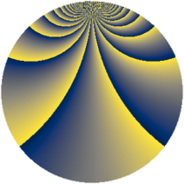# Properties

 Label 2415.2.ccLevel $2415$ Weight $2$ Character orbit 2415.cc Rep. character $\chi_{2415}(76,\cdot)$ Character field $\Q(\zeta_{22})$ Dimension $1280$ Sturm bound $768$

# Related objects

## Defining parameters

 Level: $$N$$ $$=$$ $$2415 = 3 \cdot 5 \cdot 7 \cdot 23$$ Weight: $$k$$ $$=$$ $$2$$ Character orbit: $$[\chi]$$ $$=$$ 2415.cc (of order $$22$$ and degree $$10$$) Character conductor: $$\operatorname{cond}(\chi)$$ $$=$$ $$161$$ Character field: $$\Q(\zeta_{22})$$ Sturm bound: $$768$$

## Dimensions

The following table gives the dimensions of various subspaces of $$M_{2}(2415, [\chi])$$.

Total New Old
Modular forms 3920 1280 2640
Cusp forms 3760 1280 2480
Eisenstein series 160 0 160

## Trace form

 $$1280q - 8q^{2} - 120q^{4} - 24q^{8} + 128q^{9} + O(q^{10})$$ $$1280q - 8q^{2} - 120q^{4} - 24q^{8} + 128q^{9} - 192q^{16} + 8q^{18} + 8q^{23} - 128q^{25} + 24q^{29} - 48q^{32} - 32q^{35} + 164q^{36} + 88q^{43} + 44q^{44} - 32q^{46} + 4q^{49} + 36q^{50} + 88q^{51} - 220q^{56} + 188q^{58} - 72q^{64} + 72q^{71} + 24q^{72} - 44q^{74} - 24q^{77} - 16q^{78} + 88q^{79} - 128q^{81} + 8q^{85} - 660q^{88} + 800q^{92} - 32q^{95} - 80q^{98} + O(q^{100})$$

## Decomposition of $$S_{2}^{\mathrm{new}}(2415, [\chi])$$ into newform subspaces

The newforms in this space have not yet been added to the LMFDB.

## Decomposition of $$S_{2}^{\mathrm{old}}(2415, [\chi])$$ into lower level spaces

$$S_{2}^{\mathrm{old}}(2415, [\chi]) \cong$$ $$S_{2}^{\mathrm{new}}(161, [\chi])$$$$^{\oplus 4}$$$$\oplus$$$$S_{2}^{\mathrm{new}}(483, [\chi])$$$$^{\oplus 2}$$$$\oplus$$$$S_{2}^{\mathrm{new}}(805, [\chi])$$$$^{\oplus 2}$$